Informacje o czasopiśmieFormat
Czasopismo
eISSN
2444-8656
Pierwsze wydanie
01 Jan 2016
Częstotliwość wydawania
2 razy w roku
Języki
AngielskiOtwarty dostęp

# Data Forecasting of Air-Conditioning Load in Large Shopping Malls Based on Multiple Nonlinear Regression

###### Przyjęty: 19 Mar 2022
Informacje o czasopiśmieFormat
Czasopismo
eISSN
2444-8656
Pierwsze wydanie
01 Jan 2016
Częstotliwość wydawania
2 razy w roku
Języki
Angielski

This article applies multiple nonlinear regression methods to establish a forecasting model for the load characteristics of air conditioning in shopping malls at different times. Based on Python data, determine the functional relationship of refrigerant parameters concerning pressure and temperature. The article uses kernel smoothing estimation technology to calculate the room temperature probability density distribution of users participating in DLC to characterize the user's comfort. The article's research results show that the average error between the regression analysis results of refrigerant parameters and the reference value is within 1%. This model is suitable for medium and long-term load forecasting. It has high prediction accuracy for the sudden change trend with a turning point.

#### MSC 2010

Introduction

As central air-conditioning is an important infrastructure and main energy-consuming equipment in public buildings, we must adopt reasonable operation adjustment methods to improve energy efficiency. As the basis for optimizing air-conditioning operation, air-conditioning load forecasting is also the key to the efficient and economical operation of cold storage air-conditioning systems. With the continuous progress and development of load forecasting methods, there are now many different air conditioning load forecasting methods. In practical applications, each forecasting method cannot meet the current project due to its limitations and applicable conditions . This article intends to analyze and compare several typical air-conditioning load forecasting methods based on actual engineering. The purpose is to meet the engineering practicability of air-conditioning load forecasting. Since there are many forecasting methods, we select five typical methods of linear regression, nonlinear regression, exponential smoothing, grey forecasting, and artificial neural network from different forecasting methods to study the cooling load forecast of central air conditioning. Modeling simulation analysis compares the characteristics and practicability of the above five typical forecasting methods. We expect to obtain a guiding method for air-conditioning system load forecasting in practical engineering applications.

Basic problems of load forecasting
Main factors affecting load

The cooling load of the central air-conditioning system is a typical nonlinear model with random characteristics such as slow time-varying, multi-interference, and uncertainty . Air-conditioning load forecasting mainly relies on various interference factors that affect the air-conditioning cooling load to predict the air-conditioning cooling load at a certain time or within a certain period in the future.

Studies have shown that the degree of interference from outdoor meteorological factors to the cooling load of air conditioning is in order of outdoor dry bulb temperature, outdoor solar radiation, outdoor relative humidity, outdoor wind speed, weather conditions, and day of the week . Therefore, three outdoor interference factors, outdoor dry bulb temperature, humidity, and total radiation, are selected as the interference input in this experiment. The article combines the hourly air-conditioning daily load and weather data of a certain building from June 1 to September 30 provided by the Shurisi competition platform for modeling and forecasting. We model the first 2905 sets of data as learning objects. Output the comparative data of the forecasted load and actual load on the last day, September 30, to analyze the characteristics of the forecasting method.

Evaluation index of prediction accuracy

Different assessment indicators can reflect different focuses of load forecasting. Because this article predicts the short-term cooling load of the building, we use the method of statistical probability analysis to investigate the specific daily load forecast accuracy and the percentage of qualified daily points . Among them, the daily load accuracy rate focuses on the overall characterization of the load forecast error. The percentage of daily pass points focuses on the investigation of single-point load forecast errors. The calculation method for the accuracy of daily load forecasting is as follows: $Ak=(1−1n∑i−1nEi2)×100%$ {A_k} = \left({1 - \sqrt {{1 \over n}\sum\nolimits_{i - 1}^n {E_i^2}}} \right) \times 100\% $Ei=|LFi−LRi|LRi×100%$ {E_i} = {{\left| {{L_{Fi}} - {L_{Ri}}} \right|} \over {{L_{Ri}}}} \times 100\%

Ak is the accuracy of daily load forecasting. LFi, LRi is the predicted value and actual value of the load respectively. n is the total number of daily load forecast points (24 points). Ei is the relative forecast error of a single point load. i = 1, 2,…, 24. For a single point load, the point is the qualified point when the relative prediction error Ei ≤ 3% is. The calculation method of the percentage of daily pass points is shown in formula (3): $Ri=N124×100%$ {R_i} = {{{N_1}} \over {24}} \times 100\%

N1 is the number of qualified points in the predicted load at 24 o’clock each day. The forecast date of Ri ≥ 97% is the qualifying date.

Mathematical Statistics Forecasting Method

The accuracy of load forecasting is directly related to the mathematical model of building load. With the continuous development of modeling and simulation technology, load forecasting has made great progress . With the gradual deepening of theoretical research, the method of load forecasting is constantly improving. This article first introduces four typical forecasting methods based on mathematical statistics and analyzes the characteristics of different forecasting methods when the research object remains unchanged.

Multiple linear regression

The linear regression method uses regression analysis to determine the relationship between input and output variables . It is a method based on mathematical-statistical analysis, and linearity means that the relationship between input and output variables is composed of straight lines. Its expression form is y = βi xi + e.

In this experiment, three main factors that impact the cooling load of air-conditioning were selected. There are three inputs in the regression model, namely dry bulb temperature, radiation intensity and humidity. In turn, x1, x2, x3 is the input variable, β1, β2, β3, β4 is the input parameter, and y is the output variable, that is, the air-conditioning load. The experiment obtains the linear mathematical relationship shown in formula (4) and uses it in MATLAB simulation to obtain the graph shown in Fig. 1.

$y=β1+β2x1+β3x2+β4x3$ y = {\beta _1} + {\beta _2}{x_1} + {\beta _3}{x_2} + {\beta _4}{x_3}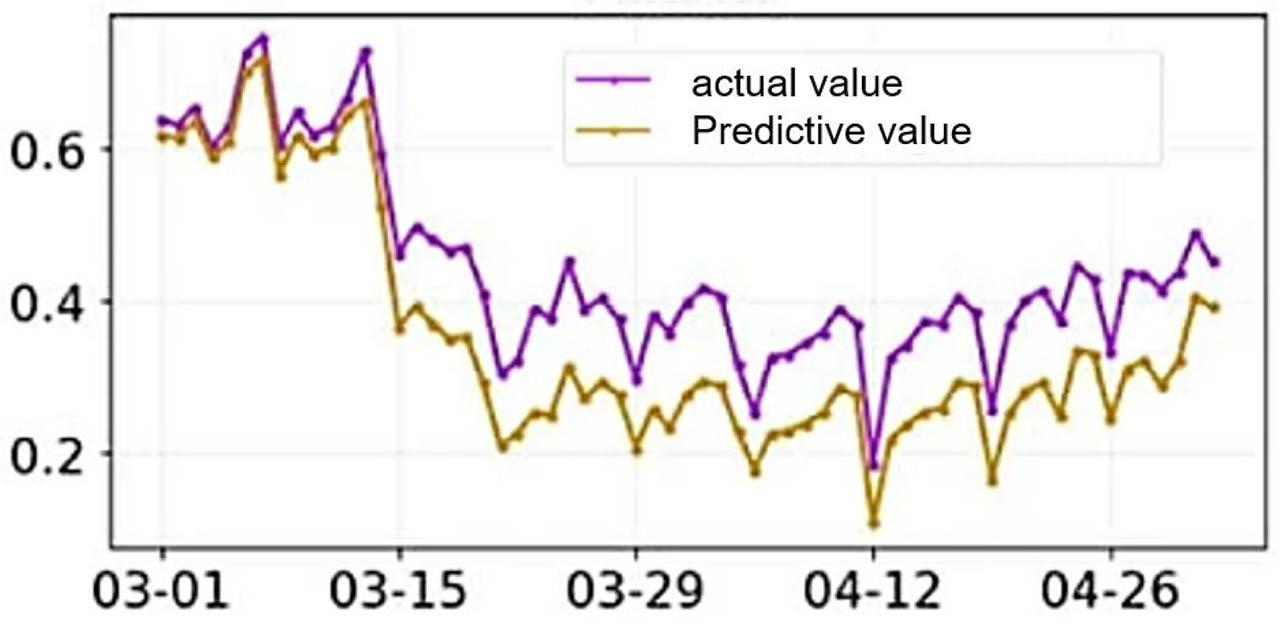The linear regression prediction value-actual value comparison chart of the measured building cooling load

The parameter values of multiple linear regression are obtained by simulation, β1 = − 7941.88, β2 = 316.20, β3 = 25.42, β4 = 3.17. We compare the predicted value with the actual value in Excel to calculate the relative error Ei. Substituting it into equations (1) and (3), the accuracy rate of daily load forecasting is calculated as A1 = −1274.9%, and the percentage of qualified daily points is R1 = 0.0%.

Multiple nonlinear regression

If the output variable of the regression model is one or more functional forms of the input variable, then the regression model is shown in the form of a curve on the graph. This type of model is called nonlinear regression . According to the number of input variables, the nonlinear regression model is divided into two types: one yuan and multiple. In this experiment, as shown in formula (5), the multivariate nonlinear cubic function is used for modeling. Substitute the input and output quantities and simulate in MATLAB to get the comparison curve shown in Figure 2.

$y=β1x13+β1x22+β1x3$ y = {\beta _1}x_1^3 + {\beta _1}x_2^2 + {\beta _1}{x_3}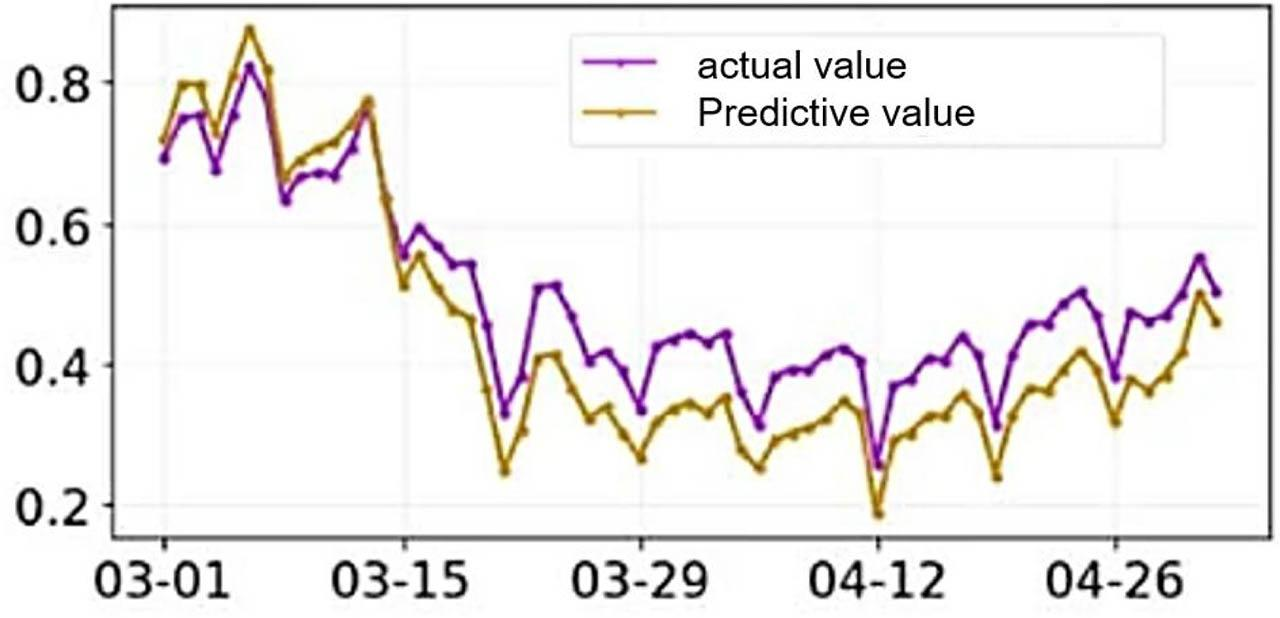The nonlinear regression prediction value-actual value comparison chart of the measured building cooling load

The parameter value β1 = 0.14, β2 = −0.05, β3 = 1.99 of multiple nonlinear linear regression is obtained by simulation. We compare the predicted value with the actual value in Excel to calculate the relative error Ei. Substituting it into equations (1) and (3), the accuracy rate of daily load forecasting is calculated as A2 = − 164.9%, and the percentage of qualified daily points is R2 = 0.0%.

Exponential smoothing

Exponential smoothing forecasts are by using raw load data as input. A method of introducing a weighting factor into the original data to obtain the predicted data. This belongs to the category of time series forecasting . The basic idea of the exponential smoothing forecasting method is that the forecasted value is a weighted sum of historical data. We give different weights to different historical data. The new data is given a larger weight, and the old data is given a smaller weight. In this experiment, to make the prediction result more accurate, the three-time exponential smoothing method is used for prediction. Its principle formula is as follows: $St(1)=αy+(1+α)St−1$ S_t^{\left(1 \right)} = \alpha y + \left({1 + \alpha} \right){S_{t - 1}} $St(2)=αSt(1)+(1−α)St−1(2)$ S_t^{\left(2 \right)} = \alpha S_t^{\left(1 \right)} + \left({1 - \alpha} \right)S_{t - 1}^{\left(2 \right)} $St(3)=αSt(2)+(1−α)St−1(3)$ S_t^{\left(3 \right)} = \alpha S_t^{\left(2 \right)} + \left({1 - \alpha} \right)S_{t - 1}^{\left(3 \right)}

When the original data is a stationary series, the value range of the parameter α is 0.1~0.3. When the data fluctuates greatly, the value range of the parameter α is 0.6–0.9. Therefore, the parameter α selected in the experiment is 0.9 to obtain the comparison curve, as shown in Figure 3. The accuracy rate of the daily load forecast is calculated, and the percentage of daily pass points is R3 = 12.5%.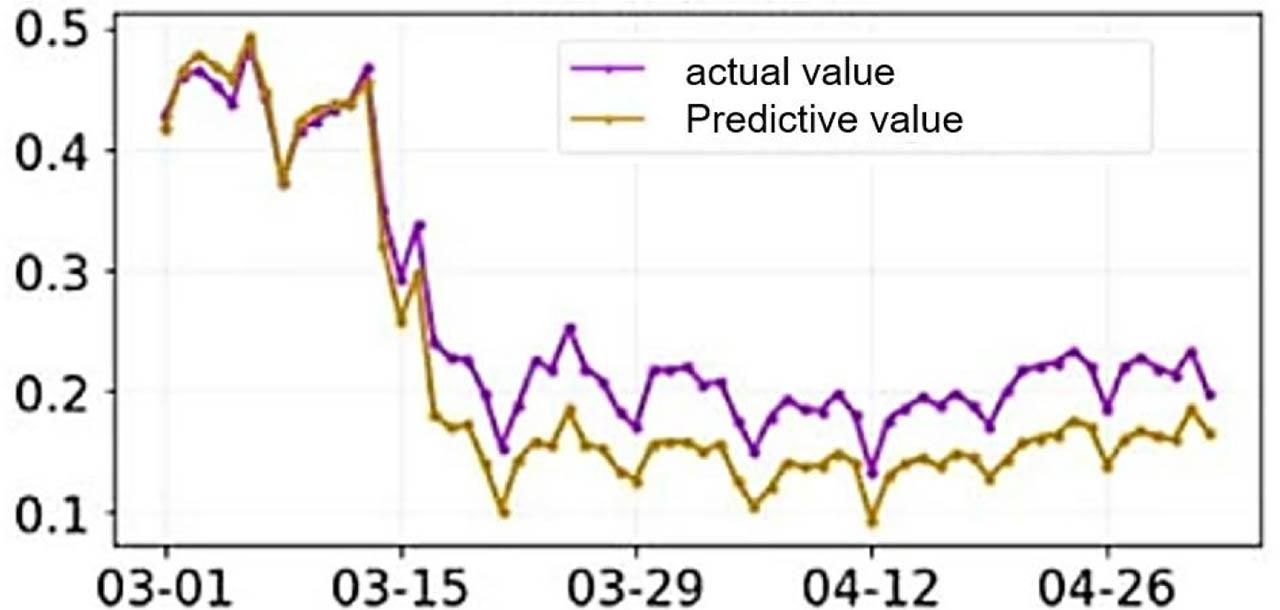Comparison of the smoothed prediction value-actual value of the cooling load index of the tested building on September 30
Grey prediction

Grey forecasting treats discrete data scattered on the time axis as a set of continuously changing sequences. The anonymous information in the gray system is weakened . At the same time, we strengthen the influence of known factors to construct a continuous differential equation with time as a variable. The parameters of gray prediction are determined by mathematical methods to achieve the purpose of prediction. It has the advantages of fewer data samples, a good short-term forecasting effect, and a simple calculation process.

Our model for predicting the gray system is called the gray model (GM). The general expression is the GM(n,x) model. Its specific meaning is to use n-order differential equations to model x variables.

The GM(1,4) model is used in this experiment. That is, the first-order differential equation is used to build a model for 4 variables. The comparative data was obtained by calculation (Figure 4). The calculated daily load forecast accuracy rate is A4 = 1.6%, and the daily pass point percentage is R4 = 0.0%.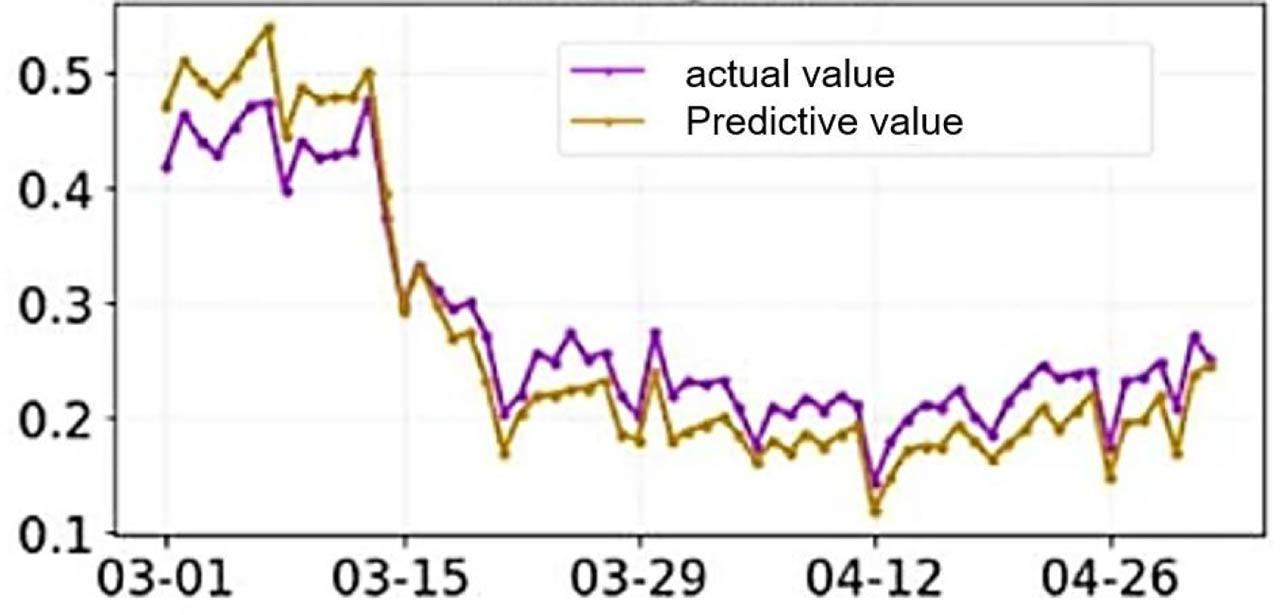Gray prediction value-actual value comparison chart of the cooling load of the tested building
BP neural network

In this experiment, the first 2905 groups of experimental data are selected as the training set for training. We use the last 24 sets of data as test samples to make predictions. The experiment uses a three-layer feedforward network. Determine the input layer variables like the actual load value, dry bulb temperature, radiation intensity, and humidity. The number of neurons in the input layer is n = 4. The number of neurons in the output layer is m = 1. We take a = 10 to get the number of neurons in the hidden layer $l=m+n+a=12$ l = \sqrt {m + n} + a = 12 .

Before entering the experimental data, the data should be normalized first to achieve comparability between data indicators. The normalization processing of neural networks generally has a variable value between [− 1, 0],[− 1,1] and [1, 0]. This is done to weaken the impact of some variables on the model when the value is large. In this experiment, the variable's value was converged to eliminate larger errors and obtain more accurate experimental results. After normalizing the data, the neural network is built. We create a BP neural network with input layer node 4, hidden layer node 12, and output node 1. Set the maximum number of iteration steps to 1000, set the learning rate to 0.05, and set the learning target to 0.000000001 (Figure 5). Then enter the data and start learning.

According to the calculation (Figure 6), the accuracy rate of daily load forecast is A5 = 99.96%, and the percentage of qualified daily points is R5 = 100%.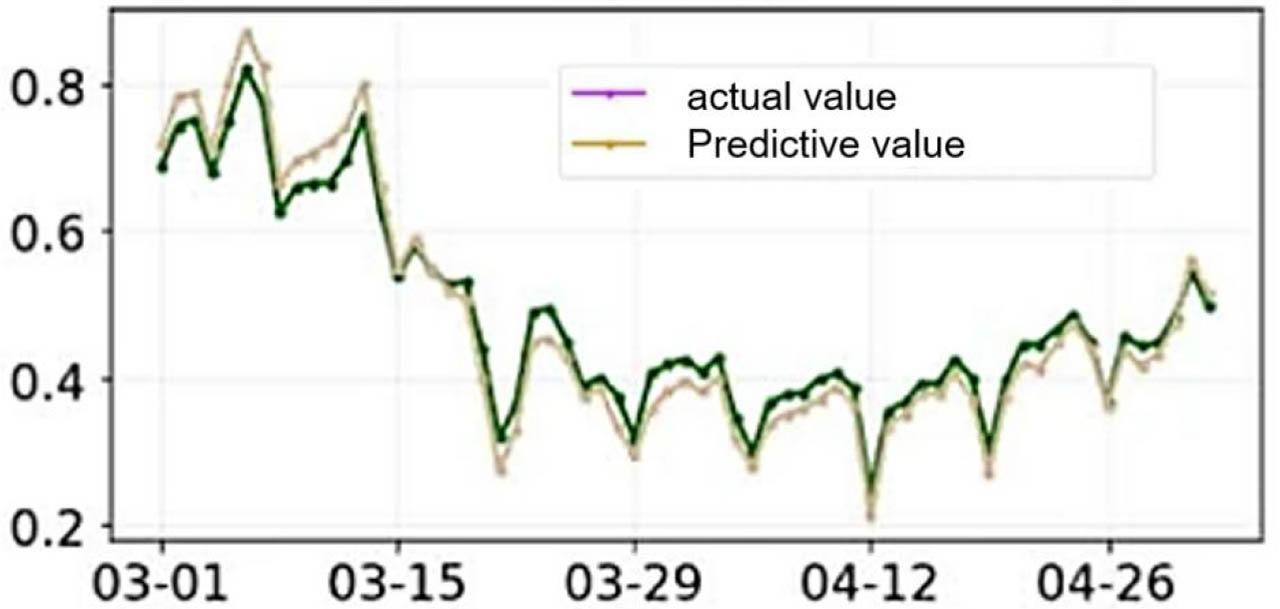Neural network prediction value-actual value comparison chart of the tested building cooling load
Discussion

This experiment is based on the historical weather data and air conditioning cooling load data of a building from June 30 to September 29 to model, learn, predict, and output the air conditioning cooling load data for the whole day on September 30. This is short-term load forecasting. After simulation in MATLAB, the prediction results output by different prediction methods are obtained. Make a unified comparison in Table 6 and analyze the characteristics of each method.

Comparison table of forecasting methods.

Method of prediction Prediction accuracy Response speed/s Input variable requirements Ease of modeling Applicability
Linear regression A1=−1274.9% 4.57 Influencing factors and historical load easy Linear model
R1=0%
Nonlinear regression A2=−164.9% 1.49 Influencing factors and historical load Easier Nonlinear model
R2=0%
Exponential smoothing A3=50.7% 0.78 Influencing factors and historical load Harder Arbitrary model
R3=12.5%
Grey forecast A4=1.6% 0.46 Historical load easy Linear model
R4=0%
BP neural network A5=99.96% 7.54 Influencing factors and historical load difficult Arbitrary model
100%

1) The accuracy of the linear regression prediction method is very low. This is because the load model of the air conditioner is not linear. Since the linear regression model connects two adjacent prediction points in a linear relationship, points with larger errors and slower response speed are prone to appear in the modeling process. The response speed of linear regression prediction will be longer and longer, and the single point error will be bigger and bigger.

2) The accuracy of the nonlinear regression prediction method is also low. Although the nonlinear model is a curve connecting the adjacent points of load forecasting, the learning ability of the nonlinear regression model is poor. The model is likely to be inapplicable because the air conditioning load changes affected by weather factors are more different than the previous day. Therefore, nonlinear regression prediction also has large errors for load models with large fluctuations in load changes. However, the response speed and accuracy of nonlinear prediction are improved compared with linear regression prediction.

3) The exponential smoothing method obviously has higher accuracy and fast response speed than the previous two forecasting methods. Exponential smoothing considers the weight of the load around the forecast day and the recent load trend, so this forecasting method can more reasonably reflect the changing trend of the load. And every time it is smoothed, the accuracy of the prediction results will be higher . This method is suitable for low-precision medium and long-term load forecasting. Grey forecast modeling is very easy and only needs historical load data to model, so the calculation and response are fast. But similar to linear regression forecasting, gray forecasting is also suitable for linear short-term load forecasting.

4) BP neural network is the prediction method with the highest prediction accuracy. Its modeling process is complicated, and its response speed is prolonged, so its forecasting accuracy is very high for short-term and medium-term load forecasting. Its response speed is also within the tolerance range of general engineering applications. It can be seen that the proportion of neural networks in practical applications is also very large.

Conclusion

Choosing a suitable forecasting method for load forecasting is of great significance in actual engineering. It is necessary to comprehensively consider the actual air-conditioning load time length, accuracy requirements, response speed, data requirements, and modeling difficulty to select a load forecasting method suitable for the actual project to achieve the best forecasting effect. The neural network method has a slower response speed but higher forecast accuracy, and it is suitable for short-term load forecasting with higher accuracy requirements. The seasonal exponential smoothing method has faster response speed and higher accuracy, and better engineering application value.

#### Comparison table of forecasting methods.

Method of prediction Prediction accuracy Response speed/s Input variable requirements Ease of modeling Applicability
Linear regression A1=−1274.9% 4.57 Influencing factors and historical load easy Linear model
R1=0%
Nonlinear regression A2=−164.9% 1.49 Influencing factors and historical load Easier Nonlinear model
R2=0%
Exponential smoothing A3=50.7% 0.78 Influencing factors and historical load Harder Arbitrary model
R3=12.5%
Grey forecast A4=1.6% 0.46 Historical load easy Linear model
R4=0%
BP neural network A5=99.96% 7.54 Influencing factors and historical load difficult Arbitrary model
100%

Fan, C., Liao, Y., & Ding, Y. Development of a cooling load prediction model for air-conditioning system control of office buildings. International Journal of Low-Carbon Technologies., 2019; 14(1): 70–75 FanC. LiaoY. DingY. Development of a cooling load prediction model for air-conditioning system control of office buildings International Journal of Low-Carbon Technologies. 2019 14 1 70 75 10.1093/ijlct/cty057 Search in Google Scholar

Datta, S. P., Das, P. K., & Mukhopadhyay, S. An optimized ANN for the performance prediction of an automotive air conditioning system. Science and Technology for the Built Environment., 2019; 25(3): 282–296 DattaS. P. DasP. K. MukhopadhyayS. An optimized ANN for the performance prediction of an automotive air conditioning system Science and Technology for the Built Environment. 2019 25 3 282 296 10.1080/23744731.2018.1526014 Search in Google Scholar

Zhao, Y., Zhang, C., Zhang, Y., Wang, Z., & Li, J. A review of data mining technologies in building energy systems: Load prediction, pattern identification, fault detection and diagnosis. Energy and Built Environment., 2020; 1(2): 149–164 ZhaoY. ZhangC. ZhangY. WangZ. LiJ. A review of data mining technologies in building energy systems: Load prediction, pattern identification, fault detection and diagnosis Energy and Built Environment. 2020 1 2 149 164 10.1016/j.enbenv.2019.11.003 Search in Google Scholar

Sulaiman, T., Bulut, H. & Baskonus, H. On the exact solutions to some system of complex nonlinear models. Applied Mathematics and Nonlinear Sciences., 2021; 6(1): 29–42 SulaimanT. BulutH. BaskonusH. On the exact solutions to some system of complex nonlinear models Applied Mathematics and Nonlinear Sciences. 2021 6 1 29 42 10.2478/amns.2020.2.00007 Search in Google Scholar

Li, T. & Yang, W. Solution to Chance Constrained Programming Problem in Swap Trailer Transport Organisation based on Improved Simulated Annealing Algorithm. Applied Mathematics and Nonlinear Sciences., 2020; 5(1): 47–54 LiT. YangW. Solution to Chance Constrained Programming Problem in Swap Trailer Transport Organisation based on Improved Simulated Annealing Algorithm Applied Mathematics and Nonlinear Sciences. 2020 5 1 47 54 10.2478/amns.2020.1.00005 Search in Google Scholar

Jianzhong, S. U. N., Fangyuan, W. A. N. G., & Shungang, N. I. N. G. Aircraft air conditioning system health state estimation and prediction for predictive maintenance. Chinese Journal of Aeronautics., 2020; 33(3): 947–955 JianzhongS. U. N. FangyuanW. A. N. G. ShungangN. I. N. G. Aircraft air conditioning system health state estimation and prediction for predictive maintenance Chinese Journal of Aeronautics. 2020 33 3 947 955 10.1016/j.cja.2019.03.039 Search in Google Scholar

Wei, Q., Liao, Z., Song, R., Zhang, P., Wang, Z., & Xiao, J. Self-learning optimal control for ice-storage air conditioning systems via data-based adaptive dynamic programming. IEEE Transactions on Industrial Electronics., 2020; 68(4): 3599–3608 WeiQ. LiaoZ. SongR. ZhangP. WangZ. XiaoJ. Self-learning optimal control for ice-storage air conditioning systems via data-based adaptive dynamic programming IEEE Transactions on Industrial Electronics. 2020 68 4 3599 3608 10.1109/TIE.2020.2978699 Search in Google Scholar

Li, K., Xue, W., Tan, G., & Denzer, A. S. A state of the art review on the prediction of building energy consumption using data-driven technique and evolutionary algorithms. Building Services Engineering Research and Technology., 2020; 41(1): 108–127 LiK. XueW. TanG. DenzerA. S. A state of the art review on the prediction of building energy consumption using data-driven technique and evolutionary algorithms Building Services Engineering Research and Technology. 2020 41 1 108 127 10.1177/0143624419843647 Search in Google Scholar

Bedi, G., Venayagamoorthy, G. K., & Singh, R. Development of an IoT-driven building environment for prediction of electric energy consumption. IEEE Internet of Things Journal., 2020; 7(6): 4912–4921 BediG. VenayagamoorthyG. K. SinghR. Development of an IoT-driven building environment for prediction of electric energy consumption IEEE Internet of Things Journal. 2020 7 6 4912 4921 10.1109/JIOT.2020.2975847 Search in Google Scholar

Liu, Y., Wei, X., Xiao, J., Liu, Z., Xu, Y., & Tian, Y. Energy consumption and emission mitigation prediction based on data center traffic and PUE for global data centers. Global Energy Interconnection., 2020; 3(3): 272–282 LiuY. WeiX. XiaoJ. LiuZ. XuY. TianY. Energy consumption and emission mitigation prediction based on data center traffic and PUE for global data centers Global Energy Interconnection. 2020 3 3 272 282 10.1016/j.gloei.2020.07.008 Search in Google Scholar

• #### Higher Education Agglomeration Promoting Innovation and Entrepreneurship Based on Spatial Dubin Model

Polecane artykuły z Trend MD